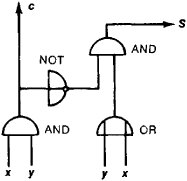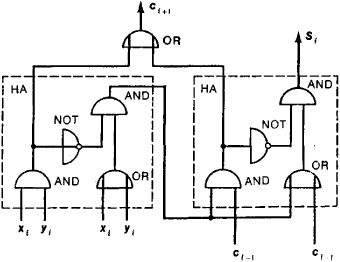Also found in: Dictionary, Thesaurus, Medical.

see viperviper,
any of a large number of heavy-bodied, poisonous snakes of the family Viperidae, characterized by erectile, hypodermic fangs. The fangs are folded back against the roof of the mouth except when the snake strikes. Vipers are distributed throughout Eurasia and Africa.
.
The following article is from The Great Soviet Encyclopedia (1979). It might be outdated or ideologically biased.

an analogue computing device whose output represents a quantity that is proportional to the sum of the input quantities, or signals. Mechanical adders are used primarily for the summing of displacements. The slide rule, for example, can be classed as a mechanical adder; it sums linear displacements. The differential gear is a mechanical adder that sums angular displacements. In electromechanical adders, the input and output quantities are mechanical displacements, and summation is carried out through use of the principles of electric circuits, particularly Kirchhoff’s laws. Electrical adders sum currents or voltages. The most common types of adders used in electronic analogue computers sum currents. To increase accuracy and raise the power and amplitude of the output signal, such adders are supplemented by operational amplifiers (seeCOMPUTING AMPLIFIER).

the basic component of the arithmetic unit of a digital computer. Adders are used to peform the operation of addition of numbers.

When decimal numbers are added digit by digit, the digits in the units digit place in the addends are added first. If the result is a one-digit number, it is entered in the units place of the final sum. If the result is a two-digit number, only the digit in the units place of this number is entered in the units place of the sum; the tens are carried—that is, the digit in the tens place is added to the digits in the tens places of the original addends. The addition operation is then carried out on the tens digits. The process is repeated for the higher order digits until the final sum is obtained. As an example, let us consider the addition of 157, 68, and 9. The digits 7, 8, and 9 are added first. Since the result is the two-digit number 24, the 4 is entered in the units place of the final sum, and the 2 is carried. The sum of 2, 5, and 6 is 13. The 3 is entered in the tens place of the final sum, and the 1 is carried. Since the sum of 1 and 1 is 2, the digit in the hundreds place in the final sum is 2. The final sum is thus 234.

When binary numbers are added digit by digit, the same process is used. The digits in the given digit place in the addends are summed, and to this result there is added the carry, if any, from the next lower place. Following the rules of addition in the binary number system, we thus obtain the digit in the given place of the final sum and the carry to the next higher place.Figure 1. Schematic of a half-adder: (x) and (y) addends, (S) sum, (c) carry to next higher digit place

A multidigit adder for digit-by-digit addition usually consists of suitably connected single-digit adding units. The simplest such unit for the addition of binary numbers is called a half-adder. A half-adder may be formed, for example, from four logic elements (Figure 1): two AND gates, an OR gate, and a NOT gate, or inverter. The circuit of a half-adder may vary in accordance with the system of logic elements used. The half-adder adds the two numbers x and y to produce the sum digit S and the carry digit c (see Table 1). To construct a multidigit adder, however, a three-digit adding unit is necessary, since three numbers must be added: the addends xi and yi and the carry ci–1 from the next lower place. The output of such a three-input adding unit is the sum Si and the carry ci+1 to the next higher place. The outputs of such an adder for the various possible inputs are shown in Table 2. An example of a three-input adder is schematically presented in Figure 2.

Table 1. Inputs and outputs of a half-adder
InputsOutputs
xySc
0000
1010
0110
1101

A number of different circuit designs and combinations of elements are used in adders. The various types of adders differ in the following ways: number system used (for example, binary adders, decimal adders, and binary coded decimal adders), number of inputs (two-input and three-input), manner of handling multidigit numbers (serial, parallel, and serial-parallel), manner

Table 2. Inputs and outputs of a three-input adder
InputsOutputs
xiYiCi – 1SiCi + 1
00000
00110
01010
01101
10010
10101
11001
11111

of organizing the summation process (combinational and accumulating), and manner of organizing the carry circuits (sequential carry, ripple-through carry, group carry, and simultaneous carry). The type of adder chosen for a particular purpose depends primarily on the system of elements used in the computer and on the speed and economy requirements. Speed is one of the most important parameters of an adder. For the sake of speed in arithmetic operations, third-generation computers use not single-digit adders but group adders, which rapidly calculate the sums and carries for a group of digit places.Figure 2. Schematic of a three-input adder consisting of two half-adders and an OR gate: (HA) half-adder, (xi) and (yi) addends, (ci – 1) carry from next lower digit place, (Si) sum, (ci + 1) carry to next higher digit place

Besides the basic operation of addition, most adders are also used for multiplication, division, and various logical operations, such as logical addition and logical multiplication.

### REFERENCES

Kartsev, M. A. Arifmetika tsifrovykh mashin. Moscow, 1969.
Kagan, B. M. and M. M. Kanevskii. Tsifrovye vychislitel’nye mashiny i sistemy. Moscow, 1973.
Presnukhin, L. N. and P. V. Nesterov. Tsifrovye vychislitel’nye mashiny. Moscow, 1974.

L. N. STOLIAROV

(computer science)
A computer device that can form the sum of two or more numbers or quantities.
(electronics)
A circuit in which two or more signals are combined to give an output-signal amplitude that is proportional to the sum of the input-signal amplitudes. Also known as adder circuit.
(vertebrate zoology)
Any of the venomous viperine snakes included in the family Viperidae.
McGraw-Hill Dictionary of Scientific & Technical Terms, 6E, Copyright © 2003 by The McGraw-Hill Companies, Inc.

1
1. a common viper, Vipera berus, that is widely distributed in Europe, including Britain, and Asia and is typically dark greyish in colour with a black zigzag pattern along the back
2. any of various similar venomous or nonvenomous snakes